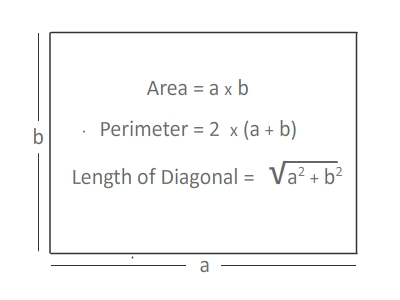# Rectangle Area, Perimeter and Length of Diagonal Calculator

Rectangle Area Calculator tool is used to calculate area, perimeter and length of the diagonal of a rectangle.#### What is Rectangle?

A rectangle is a 2D shape in, having 4 sides and 4 corners. Its two meet at . Thus, a rectangle has 4 , each measuring 90 ̊. The opposite sides of a rectangle have the same lengths and are parallel. Two sides are said to be parallel, when the distance between them remains the same at all points.

Area of Rectangle

Area of rectangle is the product of Length and Width; Area A=L X W or A=a x b.

Perimeter of Rectangle

The perimeter of a rectangle is the sum of its four sides. Since a rectangle has two equal short sides (width, b) and two equal long sides (length, a), perimeter=2a + 2b

Length of the Diagonal

A diagonal of a rectangle cuts the rectangle into two right triangles with sides equal to the sides of the rectangle and with a hypotenuse that is the diagonal. By using Pythagorous theorem, we can easily find the length of the rectangle.

Length of the Diagonal=sqrt(Length2 + Width2)

#### Fun Facts About Rectangle in Geometry

• Opposite sides are equal and parallel.
• All angles are equal to 90 degrees.
• Opposite sides are equal and parallel.
• All angles are equal to 90 degrees.
• The diagonals are equal and bisect each other.
• The intersection of the diagonals is the circumcentre. That is you can draw a circle with that as centre to pass through the four corners.